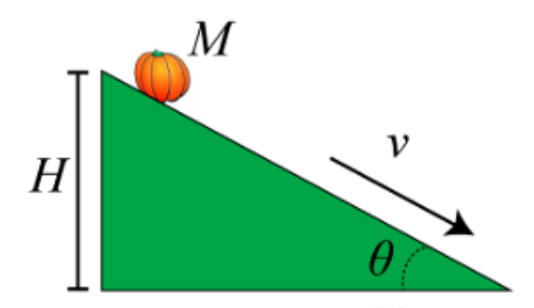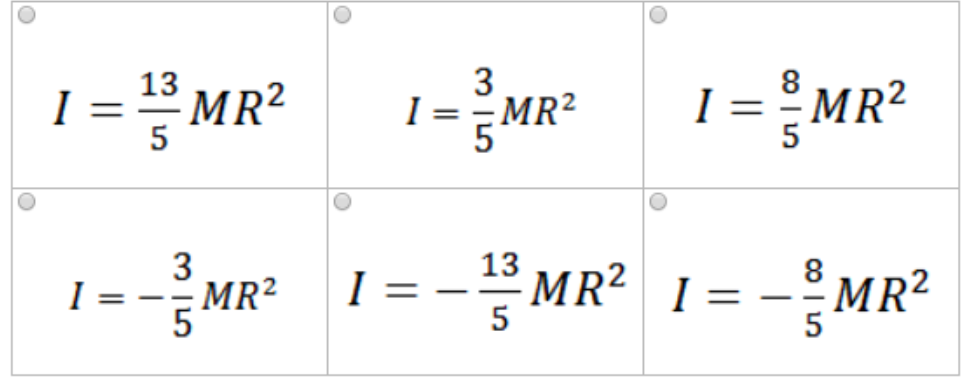# Problem: You have a pumpkin of mass M and radius R. The pumpkin has the shape of a sphere, but it is not uniform inside so you do not know its moment of inertia. In order to determine the moment of inertia, you decide to roll the pumpkin down an incline that makes an angle θ with the horizontal. The pumpkin starts from rest and rolls without slipping. When it has descended a vertical height H, it has acquired a speed of v = √(5gH/4). Find the moment of inertia I of the pumpkin in terms of M and R.

###### FREE Expert Solution

Angular velocity:

$\overline{){\mathbf{\omega }}{\mathbf{=}}\frac{\mathbf{v}}{\mathbf{r}}}$

The total kinetic energy of a rolling object:

$\overline{){\mathbf{KE}}{\mathbf{=}}\frac{\mathbf{1}}{\mathbf{2}}{\mathbit{m}}{{\mathbit{v}}}^{{\mathbf{2}}}{\mathbf{+}}\frac{\mathbf{1}}{\mathbf{2}}{\mathbit{I}}{{\mathbit{\omega }}}^{{\mathbf{2}}}}$

Energy conservation:

$\overline{){{\mathbf{U}}}_{{\mathbf{0}}}{\mathbf{+}}{{\mathbf{KE}}}_{{\mathbf{0}}}{\mathbf{=}}{{\mathbf{U}}}_{{\mathbf{f}}}{\mathbf{+}}{{\mathbf{KE}}}_{{\mathbf{f}}}}$

Potential energy:

$\overline{){\mathbf{U}}{\mathbf{=}}{\mathbf{mgh}}}$

U0 = MgH

Uf = 0 since h = 0 m

KE0 = 0 - since V = 0 m/s

KEf = (1/2)Mv2 + (1/2)Iω2 = (1/2)Mv2 + (1/2)Iv2/R2###### Problem Details

You have a pumpkin of mass M and radius R. The pumpkin has the shape of a sphere, but it is not uniform inside so you do not know its moment of inertia. In order to determine the moment of inertia, you decide to roll the pumpkin down an incline that makes an angle θ with the horizontal. The pumpkin starts from rest and rolls without slipping. When it has descended a vertical height H, it has acquired a speed of v = √(5gH/4).Find the moment of inertia I of the pumpkin in terms of M and R.